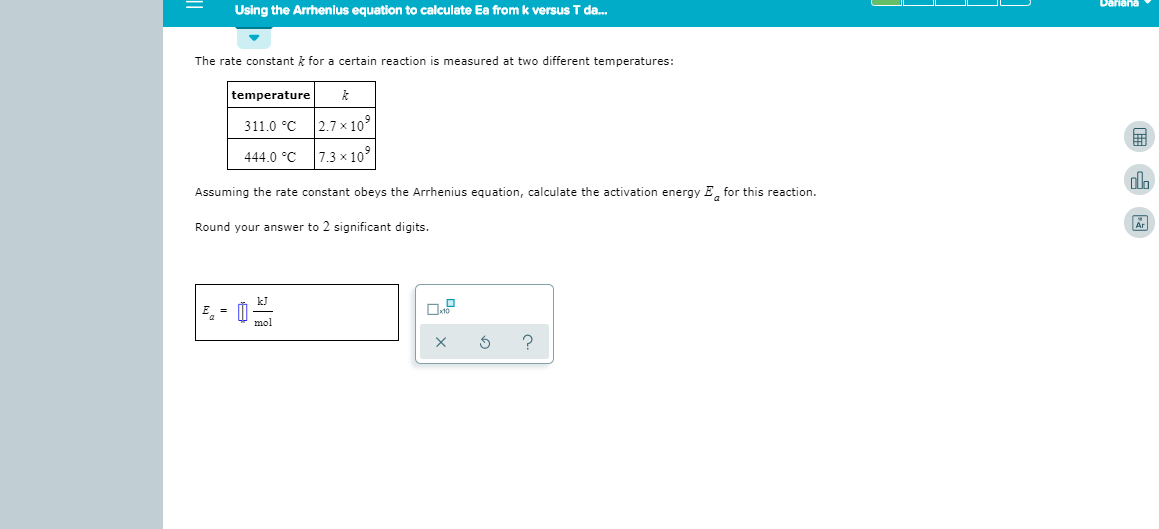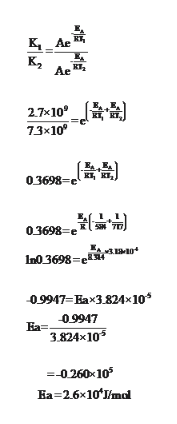# DarianaUsing the Arrhenius equation to calculate Ea from k versus T da...The rate constant k for a certain reaction is measured at two different temperatures:temperature2.7 x 109311.0 C7.3x 109444.0 CAssuming the rate constant obeys the Arrhenius equation, calculate the activation energy Efor this reactionRound your answer to 2 significant digits.kJEmolX

Question
8 viewshelp_outlineImage TranscriptioncloseDariana Using the Arrhenius equation to calculate Ea from k versus T da... The rate constant k for a certain reaction is measured at two different temperatures: temperature 2.7 x 109 311.0 C 7.3x 109 444.0 C Assuming the rate constant obeys the Arrhenius equation, calculate the activation energy Efor this reaction Round your answer to 2 significant digits. kJ E mol X fullscreen
check_circle

Step 1

According to Arrhenius Equation:

The rate of a chemical reaction is a function of the absolute temperature T of the system.

Step 2

According to Arrhenius equation taking the ratio of the two rate constants we have

The rate constant

Given,

K1=2.7 * 109

K2=27.3 * 109

T1= 311+273=584K

T2= 444 +273=717K

Step 3

Using Arrhenius equation taking the ratio...help_outlineImage TranscriptioncloseAr K2 Ae 27*10 7.3x10 03698-e 03698 In0 3698 09917-Eax3824x10* Ea=09917 3.824x10 --0.260-105 Ea-2.6x10 /ml fullscreen

### Want to see the full answer?

See Solution

#### Want to see this answer and more?

Solutions are written by subject experts who are available 24/7. Questions are typically answered within 1 hour.*

See Solution
*Response times may vary by subject and question.
Tagged in

### Chemistry